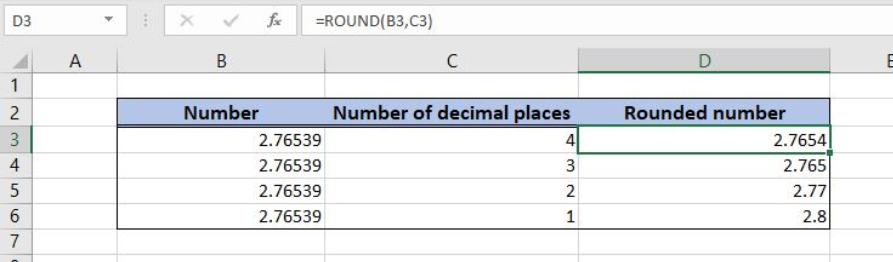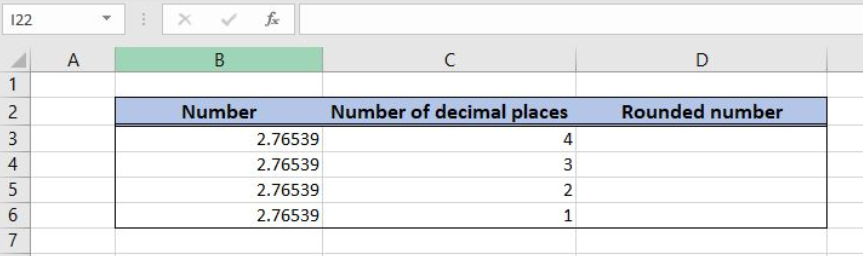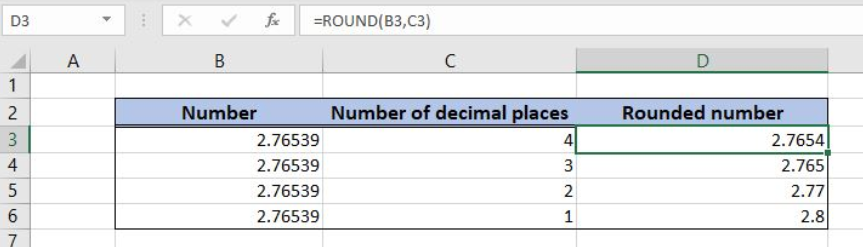Get instant live expert help with Excel or Google Sheets“My Excelchat expert helped me in less than 20 minutes, saving me what would have been 5 hours of work!”

#### Post your problem and you'll get expert help in seconds

Your message must be at least 40 characters
Our professional experts are available now. Your privacy is guaranteed.

# Round a numberRead time: 35 minutes

Excel allows us to round a decimal number to a certain number of decimal places, by using the ROUND function. This step by step tutorial will assist all levels of Excel users in rounding a decimal number.Figure 1. The result of the ROUND function

## Syntax of the ROUND Formula

The generic formula for the ROUND function is:

`=ROUND(number, num_digits)`

The parameters of the ROUND function are:

• number – a number which we want to round
• num_digits – a number of decimal places to round.

## Setting up Our Data for the ROUND Function

In column B (“Number”), we have the list of numbers and in column C (“Number of decimal places”) we have a number of decimal places for rounding. In column C (“Rounded number”), we want to get a rounded number.Figure 2. Data that we will use in the ROUND example

## Round a Number

In our example, we want to round numbers from column B to decimals in column C.

The formula in the cell D3 is:

`=ROUND(B3, C3)`

The parameter number is the cell B3, while the num_digits is C3.

To apply the ROUND function, we need to follow these steps:

• Select cell D3 and click on it
• Insert the formula: `=ROUND(B3, C3)`
• Press enter
• Drag the formula down to the other cells in the column by clicking and dragging the little “+” icon at the bottom-right of the cell.Figure 3. Using the ROUND function

When rounding the number, if the next decimal place is greater than or equal to 5, the decimal is increasing by 1. We can see that in row 3, 2.76539 is rounded to 2.7654.

Most of the time, the problem you will need to solve will be more complex than a simple application of a formula or function. If you want to save hours of research and frustration, try our live Excelchat service! Our Excel Experts are available 24/7 to answer any Excel question you may have. We guarantee a connection within 30 seconds and a customized solution within 20 minutes.

### Did this post not answer your question? Get a solution from connecting with the expert.Another blog reader asked this question today on Excelchat:## Subscribe to Excelchat.coAnother blog reader asked this question today on Excelchat: